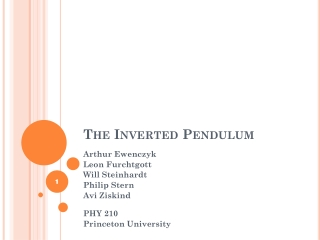DownloadDownload PresentationThe Inverted Pendulum

# The Inverted Pendulum

Download Presentation## The Inverted Pendulum

- - - - - - - - - - - - - - - - - - - - - - - - - - - E N D - - - - - - - - - - - - - - - - - - - - - - - - - - -
##### Presentation Transcript

1. The Inverted Pendulum 1 Arthur Ewenczyk Leon Furchtgott Will Steinhardt Philip Stern Avi Ziskind PHY 210 Princeton University

2. m l θ A, ω Formulating the problem • Pendulum • Mass (m) • Length (l) • Oscillating Pivot • Amplitude (A) • Frequency (ω)

3. m l θ A, ω Formulating the problem Forces Acting on the Pendulum • Gravity • Force of the pivot • [Friction]

4. m l θ A, ω Equation of Motion • Newton’s Second Law • Linear motion: • Circular motion: • Equation of Motion

5. m l θ Torques • Gravity: • Oscillating pivot: • Force: • Torque:

6. m θ θ m Equation of Motion Gravity term Pivotterm For example:

7. Physical Intuition Pivot term (dominates for large values of ω) Inertial (lab)frame Non-inertialframe (of pendulum) Butikov, Eugene. On the dynamic stabilization of an inverted pendulum. Am. J. Phys., Vol. 69, No. 7, July 2001

8. For small values of θ: Reparameterization Reparameterize: Let: Mathieu's Equation

9. Stability stable stable Regular Pendulum: Inverted Pendulum: unstable unstable Smith, Blackburn, et. al.Stability and Hopf bifurcations in an inverted pendulum. Am J. Phys. 60 (10), Oct 1992 Matlab Plot

10. (fixed) (depends on ω) Physical Region Our values of ε and δ Stability condition: (approximating A << l) ε = 0.067

11. Sample Plots

12. HOW WE BUILT IT

13. HOW WE BUILT IT

14. Why The Driver Didn’t work

15. HOW WE BUILT IT

16. HOW WE BUILT IT

17. How We Built It Some of the things we originally used to stabilize the pendulum

18. How We Built It

19. HOW WE BUILT IT

20. DATA AQUISITION

21. Data Acquisition

22. DATA AQUISITION

23. Data Speed 1 (8 Hz) Speed 2 (13 Hz) Speed 3 (23 Hz) Speed ~2.8 (22 Hz) 25

24. DATA AQUISITION 1415 RPM = 23.58 Hz

25. Problems we had Finding right amplitude and frequency Finding right equipment Stabilizing apparatus Getting apparatus to right frequency Soldering 27

26. Thank You • Professor Page • Wei Chen • PHY 210 Colleagues • Sam Cohen • Mike Peloso